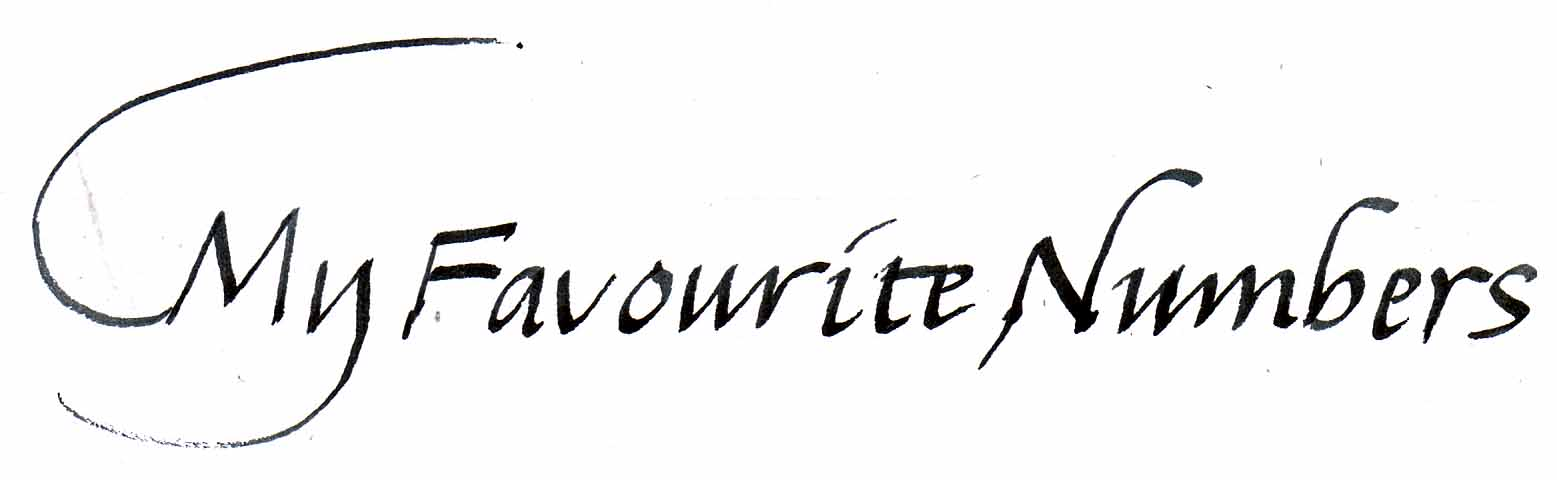James Bryant...y favourite numbersBELOW are some interesting numbers that I've collected over the years. First let me explain the notation used as some of the numbers are very large and to write them out in full with all the noughts isn't practicable. Instead I'll use the scientific notation to save space, so bear with me (it's not rocket science, trust me). Take the number 1,000,000.

You can write this as106, where the base number in my example (10) is raised to the power of 6 or in layman's terms it's just 1 with 6 noughts on the end (1,000,000). Conversely, if there is a minus sign before the power then the number is divided thus 10-6 = 10 divided by 1000000 = 0.000001.

Right, now that's sorted let's look at some of my favourite numbers...

6 is the first perfect number

7 is interesting in more ways than one. There are 7 deadly sins, 7 colours of the rainbow, 7 wonders of the World and 7 days in a week. And you cannot fold a dry piece of paper in half more than 7 times - try it! It is also the number of times light will travel around the world in one second.

22 is the maximum number of pieces that you can cut a pancake with 6 slices of the knife - try it

46 In Psalm 46, the 46th word is "shake". Then count back the 46th word from the end and find "spear" Shakespeare!

216 is he number of bones in the human body

3.8.1 The words "I Love You", have 3 letters, 8 words and only 1 meaning

5,776 is about the most stars visible to the naked eye in the northern hemisphere.

12,345 is the average number of thoughts you have each day - If you're a man half of them are sexual!

25,000 mph is the escape velocity from Earth

67,000 mph is the Earth's orbit speed around the Sun (easy to verify)

220,000 ish babies born every day

pi (p) x 107 is about the number of seconds in a year (3.142 x 10,000,000)

1.76 x 109 about the number of seconds I've been alive so far (2011)

1010 is the age of the Universe in years (remember that's 1 with 10 noughts on the end = 10,000,000,000)

111,111,111 x 111,111,111 = 12,345,678,987,654,321

1016 is about the number of words spoken since the beginning of time

1023 is roughly the end of the edge of the observable universe in miles

1080 is the number of atoms in one model of the universe.

10100 The Googol

10120 is the number of possible moves in a 60 move game of Chess - that's more than there're atoms in the universe!

If you would like to see a list of all my interesting numbers collected over the years (why?) then go click the link on this picture and download the pdf:

There are 10 sorts of people, those that understand binary and those that don't

Go to my homepage